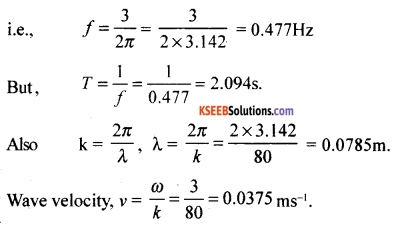# 1st PUC Physics Model Question Paper 3 with Answers

Students can Download 1st PUC Physics Model Question Paper 3 with Answers, Karnataka 1st PUC Physics Model Question Papers with Answers helps you to revise the complete Karnataka State Board Syllabus and score more marks in your examinations.

## Karnataka 1st PUC Physics Model Question Paper 3 with Answers

Time: 3.15 Hours
Max Marks: 70

General Instructions:

1. All parts are compulsory.
2. Draw relevant figure / diagram wherever necessary.
3. Numerical problems should be solved with relevant formulae.

Part – A

I. Answer the following questions: ( 10 × 1 = 10 )

Question 1.
What is physics?
Physics deals with the laws of nature and natural phenomena associated with matter and energy.

Question 2.
Give the number of significant figures in 5.300 × 103.
Four

Question 3.
What is the angle between velocity and acceleration at the peak point of a projectile projected for maximum range?
– 90° or 270°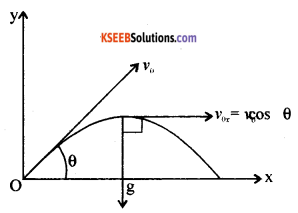Question 4.
What is the use of mechanical advantage of a lever?
The mechanical advantage of the lever is the ratio of output force to input force. By using lever with a little effect more work can be derived.Question 5.
Name the experiment to measure the value of gravitational constant.
Cavendish experiment.

Question 6.
Which property of a body is responsible for regaining its shape and size when deforming force acting on it is removed?
Elasticity.

Question 7.
Name the instrument used to measure atmospheric pressure.
Barometer.

Question 8.
What is absolute zero temperature?
Absolute zero of temperature is that temperature of a gas at which its volume and pressure become zero.
Zero kelvin = -273.15°C ≈ -273°C.

Question 9.
Define mean free path.
The average distance a molecule can travel without colliding is called the mean free path.Question 10.
What is the basis of the phenomenon of interference?
The two interfering waves should be identical and superpose at a point at small angles (travelling in the same direction) so that modification of energy takes place at the point of superposition of waves.

Part – B

II. Answer any FIVE of the following questions: ( 5 × 2 = 10 )

Question 11.
What is parallax? Mention its use.
Parallax is the change in the position of an object with respect to it background, when the object is seen from two different positions.
Parallax is used to determine long distance.

Question 12.
A car is traveling with a uniform velocity of 30ms-1. The driver applies the breaks and the car comes to rest in 10 seconds. Calculate the retardation.
u = 300 ms-1
t = 10s
v = 0
∴a = $$\frac{v-u}{t}=\frac{0-30}{10} = -3 \mathrm{ms}^{-2}$$
∴ Retardation is 3 ms-2.Question 13.
Distinguish between scalars and vectors.
Scalars:

• Scalars require only magnitude to represent them.
• Scalars require simple algebraic rules for addition or subtraction.

Vectors:

• Vectors require both magnitude and direction to represent them.
• Vectors require law of polygon of addition of vectors.

Question 14.
When is the work done negative? Give an example.
If the force and the displacement are in opposite directions, then the work is said to be negative. Work done by a force against the gravity is negative.

Question 15.
State the law of conservation of angular momentum. Illustrate with an example.
If the total external torque on a system is zero then the total angular momentum of the system is conserved (remains constant)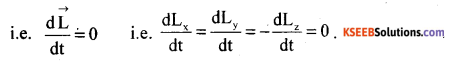Ballet dancer : Ballet dancers bring their arms closer to their body in order to increase the angular speed and decrease the moment of inertia. In order to decrease the angular speed, they stretch their arms out, thereby increasing the moment of inertia.
Thus I1ω1 = I2ω2 = L = constant.

An acrobat: In the coiled position of the body, the moment of inertia decreases and this helps the acrobat perform 2 or 3 somersaults before gravity takes over. In the stretched position moment of inertia increases and almost the turning effect becomes zero.

Question 16.
Mention the uses of polar satellites?

• Polar satellites can view polar and equatorial regions at close distances with good resolutions.
• Polar satellites are used for remote sensing, meteorology and environmental studies of the Earth.

Question 17.
State and explain zeroth law of thermodynamics.
If two thermodynamic systems are in thermal equilibrium with third individually, then the systems are said to be in mutual thermal equilibrium with each other.
If TA = TC , TB = TC then TA = TB. where ‘T’ represents the temperatures and A, B, C represent the thermodynamic systems.Question 18.
On an average a human heart is found to beat 75 times in a minute. Calculate its frequency and time period.
No. of beats = 75
Time taken = 1 minute = 60 second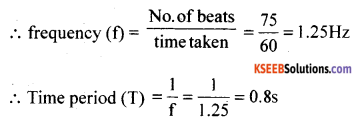Part – C

III. Answer any FIVE of the following questions: ( 5 × 3 = 15 )

Question 19.
State and explain triangle law of vector addition.
If two vectors are represented by the two sides of a triangle taken in order then their resultant is represented by the closing side in the opposite order.

Question 20.
Prove that for a particle in rectilinear motion under constant acceleration, the change in kinetic energy of the particle is equal to the work done on it by the net force?
The difference of PE. or K..E. required by a body at two different positions is equal to the amount of work done.
$$\int_{k_{i}}^{k_{i}} d(K E)=\frac{1}{2} m\left(v^{2}-u^{2}\right)$$
= mas = Fs
= work done
i. e. W = Kf – Ki.

Question 21.
Draw the stress verses strain graph for a metallic wire stretched up to fracture point. Define the terms proportional limit and fracture point.
Statement: The ratio of stress to strain is a constant for a material within the elastic limit.Modulus of elasticity = $$\frac{\text { Stress }}{\text { Strain }}$$

Within the elastic limit, stress v/s strain is a straight line ‘A’ is the elastic limit upto which Hooke’s law is applicable. Beyond ‘B’ the yielding point, the wire extends but does not return to the initial state when the deforming force is removed. ‘F’ is the breaking point. ‘EF’ allows the material to be malleable and ‘DE’, ductile.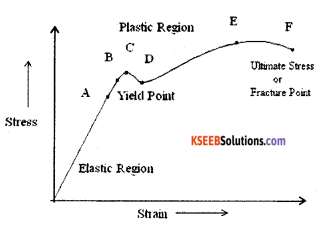Question 22.
Find the torque of a force $$7 \hat{i}+3 \hat{j}-5 \hat{k}$$ about the origin. The force acts on a particle whose position vector is $$\hat{i}-\hat{j}+\hat{k}$$.
$$\overrightarrow{\mathrm{F}}=7 \hat{i}+3 \hat{j}-5 \hat{k} ; \vec{r}=\hat{i}-\hat{j}+\hat{k}$$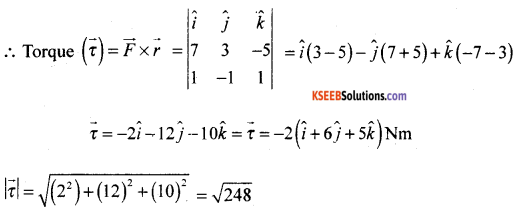∴τ = 15.74 Nm

Question 23.
What is capillary rise? Write an expression for it. Explain the terms.
A rise in a liquid above the level of zero pressure due to a net upward force produced by the attraction of the water molecules to a solid surface.

Question 24.
Write any three assumptions of kinetic theory of gases.

1. Collisions of molecules on the walls are perfectly elastic.
2. The size of the molecules are neglected when compared to the volume of the container.
3. The contribution to the net pressure due to inter-molecular collisions is neglected.
4. The root mean square value of speed is the average of velocities taken in all the three directions.

Question 25.
State and explain the law of thermal conduction.
The quantity of heat conducted during the steady state is directly proportional to the,
(i) Area of cross-section of a conductor.
(ii) Temperature difference between the two points or ends (θ1 – θ2).
(iii) Time of passage of heat (t) and inversely proportional to the thickness or distance between the two ends of the conductor (d).
i.e., Q = $$\frac{\mathrm{KA}\left(\theta_{1}-\theta_{2}\right) \mathrm{t}}{\mathrm{d}}$$
where ’K’ is thermal conductivity of the material of the conductor.
The SI unit of thermal conductivity is Wm-1 K-1.Question 26.
Mention any three differences between standing waves and progressive waves.

1. Progressive waves transfer energy, whereas stationary waves do not.
1. No particles of the medium are in a state of rest in a PW but in a SW, the particles at nodes will be at rest.
2. The particle velocities will remain the same for a given displacement in a PW, whereas in a SW, particles at nodes will have zero speed and at antinodes, particles have maximum speed.
3. y = f(x, t) for a PW and y = f(x) g(t) for a SW.
4. Distance between two points at which particles have same state of vibration is called wavelength in a PW whereas distance between two consecutive nodes or antinodes is half the wavelength.

Part – D

IV. Answer any TWO of the following questions : ( 2 × 5 = 10 )

Question 27.
What is velocity – time graph. Derive the equation of motion: v2 – v02 = 2ax.
Let ‘v0’ be the initial velocity of a particle describing uniform accelerated motion ‘a’.
Slope of the line AB = a = tanθ = where BC = v – v0 and AC = t
i.e., a = $$\frac{v-v_{0}}{t}$$ ……….(1)
But area of trapezium = x = 1/2 × OD × (OA + BD)
i.e., x = 1/2 t(v0 + v)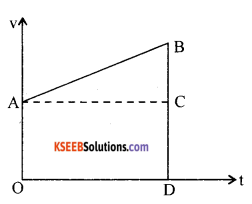From(1), t = $$\frac{v-v_{0}}{a}$$
Hence (2) may be written as 2s = $$\left(\frac{v-v_{0}}{a}\right)$$(v + v0)
∴v2 – v02 = 2ax.Question 28.
Derive the expression for maximum safe speed of a vehicle on a banked road in circular motion.
From the figure, ‘θ’ is the angle of elevation of outer edge over the inner edge. ‘R’ is the normal reaction force.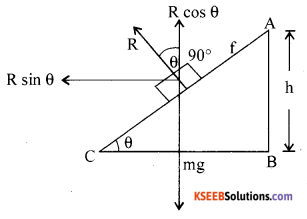R sinθ = $$\frac{m v^{2}}{r}$$………(1)
Rcosθ = mg  ………(2)
(1) ÷ (2) gives
tanθ = $$\frac{v^{2}}{r g}$$
∴vmax = $$=\sqrt{r g \tan \theta}$$

Question 29.
What is elastic collision? Obtain the expression for final velocities of two bodies undergoing elastic collision in one dimension.
A collision in which linear momentum and kinetic energy of the interacting system are conserved, is known as an elastic collision.
From the law of conservation of linear momentum,
m1 v1i + m2 v2i = m1v1f + m2v2f ……….(1)
From the law of conservation of total kinetic energy,
$$\frac{1}{2} m_{1} v_{1 i}+\frac{1}{2} m_{2} v_{2 i}=\frac{1}{2} m_{1} v_{1 f}^{2}+\frac{1}{2} m_{2} v_{2 f}^{2}$$….(2)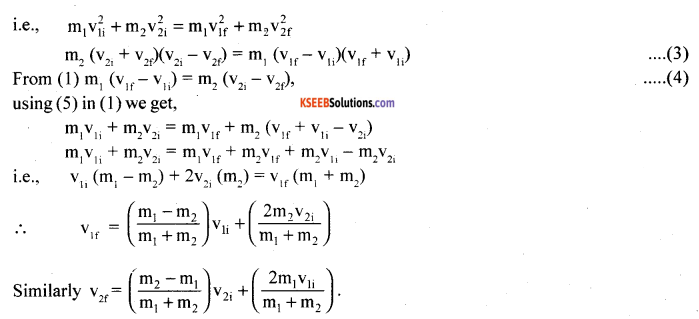V. Answer any TWO of the following questions: ( 2 × 5 = 10 )

Question 30.
What is escape speed? Obtain the expression for escape speed. What is the value of escape speed for earth?
It is the minimum speed needed for a free object to escape from the gravitational influence of a massive body.
For a satellite to escape into infinite orbit,
∆PE = PE – PE∵PE = 0
But K.E = PE.
∆PE = $$-\left(-\frac{\mathrm{GMm}}{\mathrm{R}+\mathrm{h}}\right)$$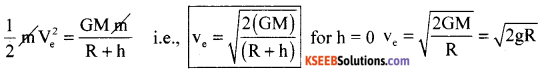It is assumed that at infinite distance PE = 0, v1 = 0.
It is a free fall of an object from the point of infinity to a point on the surface in the absence of any frictional forces.Question 31.
What is isothermal process? Obtain the expression for work done in an isothermal process.
The process in which changes in pressure and volume of a gas system takes place at constant temperature of the system, is known as isothermal process.
For an isothermal process, PV = constant.
At any intermediate stage with pressure P and change in volume from V to V + dV,
Work done dW = P dV. However for the entire process W = $$=\int_{V_{1}}^{V_{2}}$$PdV.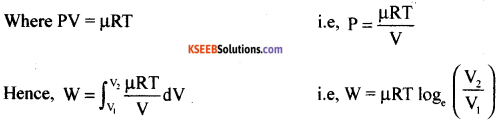For V2 > V1, W > 0 and for V2 < V1, W < 0.
In an isothermal expansion, the gas absorbs heat and does work, while in an isothermal compression, work is done on the gas by the environment and heat is released.

Question 32.
What is simple harmonic motion? Derive an expression for velocity and acceleration in simple harmonic motion.
A SHM is the simplest form of oscillatory motion in which the force on the oscillating particle or body is directly proportional to the displacement from the mean position and the force directed towards the mean position.
We know that, the displacement of the particle
y = A sin ωt
But v = $$\frac{d y}{d t}$$
∴v = $$\frac{d}{d t}$$(A sin ωt)
and acceleration,
a = $$\frac{d v}{d t}$$
∴a = $$\frac{d}{d t}$$(Aω cos ωt)
a = -ω2 A sin ωt

VI. Answer any THREE of the following questions : ( 3 × 5 = 15 )

Question 33.
A cricketer can throw a ball to a maximum horizontal distance of 100 m..How much high above the ground can the cricketer throw the same ball? And for how long the bail remains in the air in this case?
R = 100 m
Rmax = $$\frac{v^{2}}{g}$$    θ = 45°
∴v2 = Rmax x g
v2 = 100 × 9.8
v2 = 980 m2s-2 and v = 31.3 ms-1
∴the maximum height can be calculated, by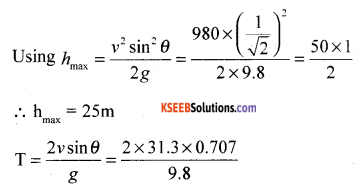T = 4.52sQuestion 34.
A stone of mass 0.25 kg tied to the end of a string is whirled round in a circle of radius 1.5 m with a speed of 40 rev./min in a horizontal plane. What is the tension in the string? W hat is the maximum speed with which the stone can be whirled around if the string can withstand a maximum tension of 200 N?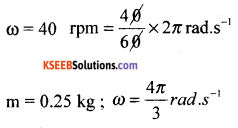Tmax = $$\frac{m v^{2}}{r}$$
Tmax = mrω2 [v = rω]
= 0.25 x 1.5 x $$\left(\frac{4 \pi}{3}\right)^{2}$$
As the string can withstand a maximum tension of 200 N.
Tmax = $$\frac{m v_{\max }^{2}}{r}$$
Tmax = $$\frac{T_{\max } \times r}{m}$$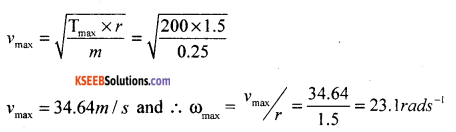Question 35.
A solid cylinder of mass 20 kg rotates about its axis with angular speed 100 rad s-1. The radius of the cylinder is 0.25 m. What is the kinetic energy associated with the rotation of the cylinder? Calculate the magnitude of angular momentum of the cylinder about its axis.
m = 20 kg
r = 0.25 m
(i) The moment of inertia of the solid cylinder,
I = $$\frac{m r^{2}}{2}$$ = $$\frac{1}{2}$$ × 20 × (0.25)
I = 0.625 kgm2
(ii) The kinetic energy of rotation, KE = $$\frac{1}{2} \mathrm{I} \omega^{2}$$
KE $$=\frac{1}{2} \mathrm{I} \omega^{2}=\frac{1}{2} \times 0.625 \times(100)^{2}$$
K.E = 3125J
(iii) Angular momentum,
L = Iω
i.e.,L = 0.625 × 100
L = 62.5 JsQuestion 36.
A brass rod of length 50 cm and diameter 3.0 mm is joined to a steel rod of the same length and diameter. What is the change in length of the combined rod at 250° C if the original lengths arc at 40.0° C? Is there a ‘thermal stress’ developed at the junction? The ends of the rod are free to expand.
(Co-efficient of linear expansion of brass = 2.0 × 105 K-1 , steel = 1.2 × 10-5 K-1).
T1 = 40°C, T2 = 250°C
∴∆T = 210°C
For brass rod, initial length (L0) = 50 cm
Let final length = L
αb = 2 × 10-5 /K
L = L0 (1 + αb ∆T) = 50 (1 + 2 × 10-5 × 210) = 50(1 + 420 × 10-5)
L = 50.21 cm
∴Increase in length (∆L) = L – L0 = 50.21 – 50
∆L = 0.21 cm
For steel rod,
Initial length (L01) = 50 cm
Let final length = L1
αs =1.2 × 10-5 K-1
∴L1 = L01 (1 + αs ∆T) = 50 (1 + 1.2 × 10-5 × 210) = 50 × 1.00252
L1 = 50.126 cm
∴increase in length (∆L1) = 50.126 – 50 = 0.126 cm
∴Total increase in length = ∆L + ∆L1 = 0.21 + 0.126 = 0.336 cmQuestion 37.
A wave travelling along a string is described by, y (x, t) = 0.005 sin (80.0 × – 3.0 t), in which the numerical constants are in St units (0.005 m, 80.0 rad m-1, and 3.0 rad s-1). Calculate (a) the amplitude, (b) the wavelength, and (c) the frequency of the wave. Also, calculate the displacement jr of the wave at a distance x = 30.0 cm and time t = 20 s?